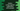# Python numpy append method explanation with example## Python numpy append method explanation with example:

Numpy append() method is used to append values to the end of an array. Using append, we can join two numpy arrays. It doesn’t modify the original array, but appends data to the end and returns one new array.

In this post, we will learn how to use numpy append with examples.

### Syntax of numpy append:

Below is the syntax of numpy append() :

``numpy.append(arr, values, axis = None)``

Here, arr - This is the original array_like variable. All values are appended to the end of its copy.

values - These are values that we are appending to the end of arr. It should be of same shape as arr excluding axis. If axis is not specified, it can be any shape and it will be flattened before use. It is a array_like variable.

axis - It is an optional value of type int. It is the axis along which values will be appended. 1 indicates axis of the first row and 0 indicates axis of the first column. If this is not given, both arr and values are flattened before use.

### Return value of numpy append:

It returns a ndarray. A newly created array is returned without modifying the original one. For axis None, it returns a flattened array.

### Example of numpy append with 1D arrays:

Let’s try append with two 1D arrays :

``````import numpy as np

first_arr = np.array([1, 2, 3, 4, 5])
second_arr = np.array([6, 7, 8, 9, 10])

result_array = np.append(first_arr, second_arr)

print(result_array)``````

Here,

• first_arr and second_arr are two numpy arrays initialized with different numbers.
• Using append, we are appending second_arr to the end of first_arr.

This program prints the below output:

``[ 1  2  3  4  5  6  7  8  9 10]``

We are not using axis here.

### Example of numpy append with 2D arrays:

#### Without any axis value:

Now, let’s try append with two 2D arrays. First of all, we will try without using axis value. It will flatten the arrays and append them:

``````import numpy as np

first_arr = np.array([[1, 2, 3], [4, 5, 6]])
second_arr = np.array([[7, 8, 9], [10, 11, 12]])

result_array = np.append(first_arr, second_arr)

print(result_array)``````

It will print:

``[ 1  2  3  4  5  6  7  8  9 10 11 12]``

As you can see here, it flatten the arrays to one dimensional array.

#### With axis = 0:

``````import numpy as np

first_arr = np.array([[1, 2, 3], [4, 5, 6]])
second_arr = np.array([[7, 8, 9], [10, 11, 12]])

result_array = np.append(first_arr, second_arr, axis=0)

print(result_array)``````

It will print:

``````[[ 1  2  3]
[ 4  5  6]
[ 7  8  9]
[10 11 12]]``````

#### With axis = 1:

``````import numpy as np

first_arr = np.array([[1, 2, 3], [4, 5, 6]])
second_arr = np.array([[7, 8, 9], [10, 11, 12]])

result_array = np.append(first_arr, second_arr, axis=1)

print(result_array)``````

It will print:

``````[[ 1  2  3  7  8  9]
[ 4  5  6 10 11 12]]``````

So, based on the value of axis, it appends the items differently.

### ValueError:

append throws ValueError if the input arrays are of different dimensions and if we are using axis. For example,

``````import numpy as np

first_arr = np.array([[1, 2, 3], [4, 5, 6]])
second_arr = np.array([7, 8, 9])

result_array = np.append(first_arr, second_arr, axis=1)

print(result_array)``````

It will throw ValueError.